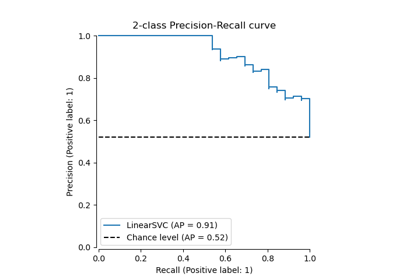# sklearn.metrics.average_precision_score¶

sklearn.metrics.average_precision_score(y_true, y_score, *, average='macro', pos_label=1, sample_weight=None)[source]

Compute average precision (AP) from prediction scores.

AP summarizes a precision-recall curve as the weighted mean of precisions achieved at each threshold, with the increase in recall from the previous threshold used as the weight:

$\text{AP} = \sum_n (R_n - R_{n-1}) P_n$

where $$P_n$$ and $$R_n$$ are the precision and recall at the nth threshold . This implementation is not interpolated and is different from computing the area under the precision-recall curve with the trapezoidal rule, which uses linear interpolation and can be too optimistic.

Note: this implementation is restricted to the binary classification task or multilabel classification task.

Read more in the User Guide.

Parameters
y_truendarray of shape (n_samples,) or (n_samples, n_classes)

True binary labels or binary label indicators.

y_scorendarray of shape (n_samples,) or (n_samples, n_classes)

Target scores, can either be probability estimates of the positive class, confidence values, or non-thresholded measure of decisions (as returned by “decision_function” on some classifiers).

average{‘micro’, ‘samples’, ‘weighted’, ‘macro’} or None, default=’macro’

If None, the scores for each class are returned. Otherwise, this determines the type of averaging performed on the data:

'micro':

Calculate metrics globally by considering each element of the label indicator matrix as a label.

'macro':

Calculate metrics for each label, and find their unweighted mean. This does not take label imbalance into account.

'weighted':

Calculate metrics for each label, and find their average, weighted by support (the number of true instances for each label).

'samples':

Calculate metrics for each instance, and find their average.

Will be ignored when y_true is binary.

pos_labelint or str, default=1

The label of the positive class. Only applied to binary y_true. For multilabel-indicator y_true, pos_label is fixed to 1.

sample_weightarray-like of shape (n_samples,), default=None

Sample weights.

Returns
average_precisionfloat

roc_auc_score

Compute the area under the ROC curve

precision_recall_curve

Compute precision-recall pairs for different probability thresholds

Notes

Changed in version 0.19: Instead of linearly interpolating between operating points, precisions are weighted by the change in recall since the last operating point.

References

1

Wikipedia entry for the Average precision

Examples

>>> import numpy as np
>>> from sklearn.metrics import average_precision_score
>>> y_true = np.array([0, 0, 1, 1])
>>> y_scores = np.array([0.1, 0.4, 0.35, 0.8])
>>> average_precision_score(y_true, y_scores)
0.83...


## Examples using sklearn.metrics.average_precision_score¶